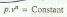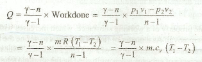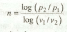# Polytropic Process

The polytropic process is also known as the general law for the expansion and compression of gases and is given by the relation:where n is a polytropic index, which may have any value from zero to infinity, depending upon the manner, in which the expansion or compression has taken place. The various equations for polytropic process may be expressed by changing the index n for Ɣ in the adiabatic process.

The heat absorbed or rejected during the polytropic process is given byNotes:

(a) When n is less than Ɣ , then heat is absorbed by the gas.
(b) When n is greater than Ɣ , then heat is rejected by the gas.
(c) The polytropic index (n) is given by